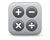# ASX bond calculator

ASX has developed a bond calculator to help you easily calculate an AGB’s yield to maturity from the traded price. Comparing the yield to maturity of different AGBs will assist in determining which AGB is the more attractive investment for your requirements.

You should make sure you’re familiar with the key terms relating to bonds before investing or using the ASX bond calculator, make sure you're familiar with the key terms.## Using the bond calculator

The ASX bond calculator has been specifically developed for bonds quoted on ASX. Once you have entered into the calculator either the market price or yield for your chosen AGB, the calculator will produce the:

• market price based on the yield to maturity;
• yield to maturity based on the market price;
• clean price (market price less accrued interest); and
• accrued interest.

If you enter the yield to maturity, the calculator will produce the market price, clean price and accrued interest.

## Understanding the key terms

• Accrued interest: the interest that has accumulated since the principal investment, or since the previous coupon interest payment date if there has been one already.
• Basis points: a basis point equals one hundredth of a percentage point (0.01%). 100 basis points equals 1%. If a bond yield has gone up by 50 basis points, it has gone up by 0.50% (eg from 4.00% to 4.50%).
• Bid/ask: the bid price is the price offered by a buyer. The ask (or offer) price is the price offered by the seller. The bid price will always be lower than the ask price, excpet when there is a trade when the prices are equal and matched.
• Clean price: the price of a bond not including any accrued interest. The clean price becomes equal to the market price immediately following a coupon payment.
• Coupon rate: the nominal rate the bond pays.
• Duration: the effective maturity of a bond and its sensitivity to changes in interest rates. When comparing two bonds, calculating duration makes it easier to tell which one to buy if each has a different coupon rate and different maturity date.
• Market price: the price of a bond, including the interest accrued on the next coupon payment. This is sometimes referred to as the ‘gross’ or 'dirty' price.
• Nominal value: the CPI-adjusted face value of Treasury Indexed Bonds (TIBs).
• Par: the face value of a bond at issue date.
• Premium and discount bonds: if a bond’s price is quoted as higher than its face value, it is called a premium bond. If a bond is quoted at less than its face value, it is known as a discount bond.
• Yield to maturity - 'YTM': the rate of return anticipated on a bond if it is held until the maturity date. YTM is considered a long-term bond yield expressed as an annual rate. The calculation of YTM takes into account the current market price, par value, coupon interest rate and time to maturity. It is also assumed that all coupons are reinvested at the same rate. Sometimes this is simply referred to as 'yield' for short.